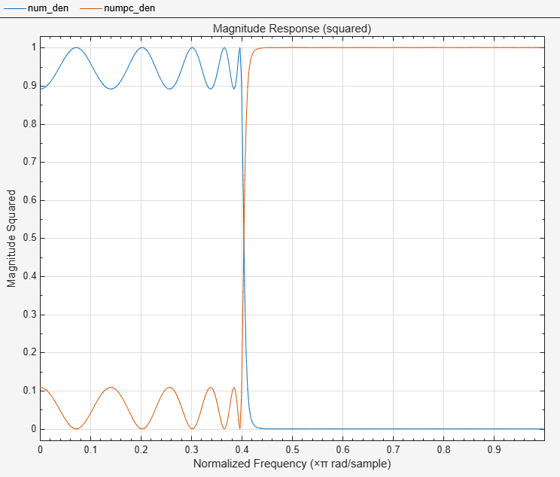# ca2tf

Convert coupled allpass filter to transfer function form

## Syntax

``[b,a] = ca2tf(d1,d2)``
``[b,a] = ca2tf(d1,d2,beta)``
``[b,a,bp] = ca2tf(d1,d2)``
``[b,a,bp] = ca2tf(d1,d2,beta)``

## Description

````[b,a] = ca2tf(d1,d2)` returns the vector of coefficients of `b` and `a`. `b` and `a` corresponds to the numerator and the denominator of the transfer function H(z), respectively, where `d1` and `d2` are real vectors corresponding to the denominators of the allpass filters H1(z) and H2(z).$H\left(z\right)=B\left(z\right)/A\left(z\right)=\frac{1}{2}\left[H1\left(z\right)+H2\left(z\right)\right]$```
````[b,a] = ca2tf(d1,d2,beta)` returns the vector of coefficients `b` and the vector of coefficients `a` corresponding to the numerator and the denominator of the transfer function H(z), respectively, where `d1` and `d2` are complex vectors and `beta` is a complex scalar.$H\left(z\right)=B\left(z\right)/A\left(z\right)=\frac{1}{2}\left[-\left(\overline{\beta }\right)\cdot H1\left(z\right)+\beta \cdot H2\left(z\right)\right]$```
````[b,a,bp] = ca2tf(d1,d2)` also returns the vector `bp` of real coefficients corresponding to the numerator of the power-complementary filter G(z), where `d1` and `d2` are real vectors.$G\left(z\right)=Bp\left(z\right)/A\left(z\right)=\frac{1}{2}\left[H1\left(z\right)-H2\left(z\right)\right]$```
````[b,a,bp] = ca2tf(d1,d2,beta)` also returns the vector of coefficients `bp` of real or complex coefficients that correspond to the numerator of the power-complementary filter G(z), where `d1` and `d2` are complex vectors and `beta` is a complex scalar.$G\left(z\right)=Bp\left(z\right)/A\left(z\right)=\frac{1}{{2}_{j}}\left[-\left(\overline{\beta }\right)\cdot H1\left(z\right)+\beta \cdot H2\left(z\right)\right]$```

## Examples

collapse all

Create a coupled allpass filter.

`[b,a] = cheby1(10,.5,.4);`

The `tf2ca` function returns the denominators of the coupled allpass filter.

`[d1,d2,beta] = tf2ca(b,a);`

Reconstruct the original and the power-complementary filters using the `ca2tf` function.

`[num,den,numpc] = ca2tf(d1,d2,beta);`

Plot the magnitude response of the original filter and the power-complementary one.

```fvtool(num,den,numpc,den,'Analysis','magnitude','MagnitudeDisplay',... 'Magnitude Squared')```## Input Arguments

collapse all

Denominator of the allpass filter H1(z), specified as a real- or complex-valued vector.

Data Types: `single` | `double`
Complex Number Support: Yes

Denominator of the allpass filter H2(z), specified as a real- or complex-valued vector.

Data Types: `single` | `double`
Complex Number Support: Yes

Complex scalar of magnitude 1.

Data Types: `single` | `double`
Complex Number Support: Yes

## Output Arguments

collapse all

Numerator coefficients of the transfer function H(z), returned as a real- or complex-valued vector.

Data Types: `double`
Complex Number Support: Yes

Denominator coefficients of the transfer function H(z), returned as a real- or complex-valued vector.

Data Types: `double`
Complex Number Support: Yes

Numerator coefficients of the power-complementary filter G(z), returned as a real- or complex-valued vector.

Data Types: `double`
Complex Number Support: Yes

 Vaidyanathan, P. P., and Sanjit K. Mitra. Robust Digital Filter Structures: A Direct Approach. IEEE Circuits and Systems Magazine 19, no. 1 (2019): 14–32. https://doi.org/10.1109/MCAS.2018.2889204.

 Vaidyanathan, P. P. Multirate Systems and Filter Banks. Prentice-Hall Signal Processing Series. Englewood Cliffs, N.J: Prentice Hall, 1993.

## Support평가판 신청